Ex 5.1

Chapter 5 Class 12 Continuity and Differentiability
Serial order wise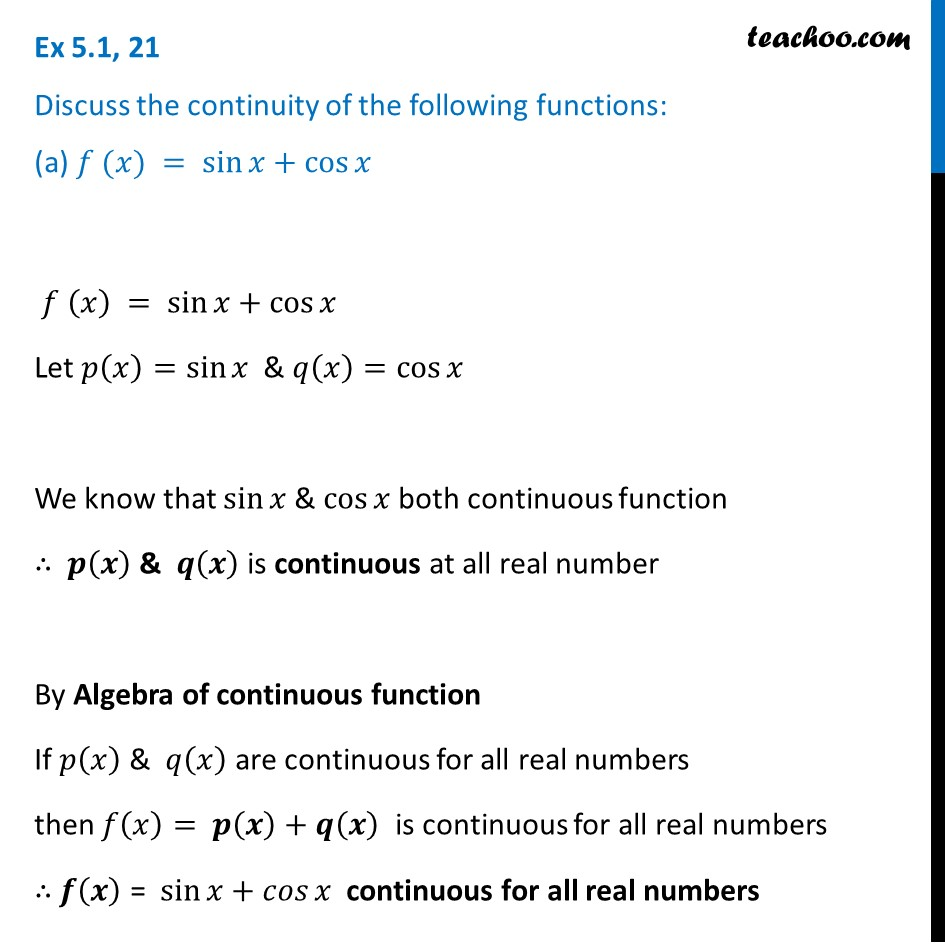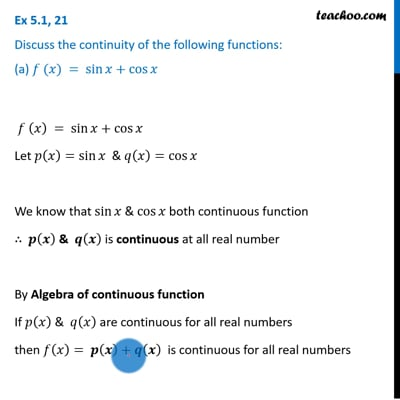This video is only available for Teachoo black users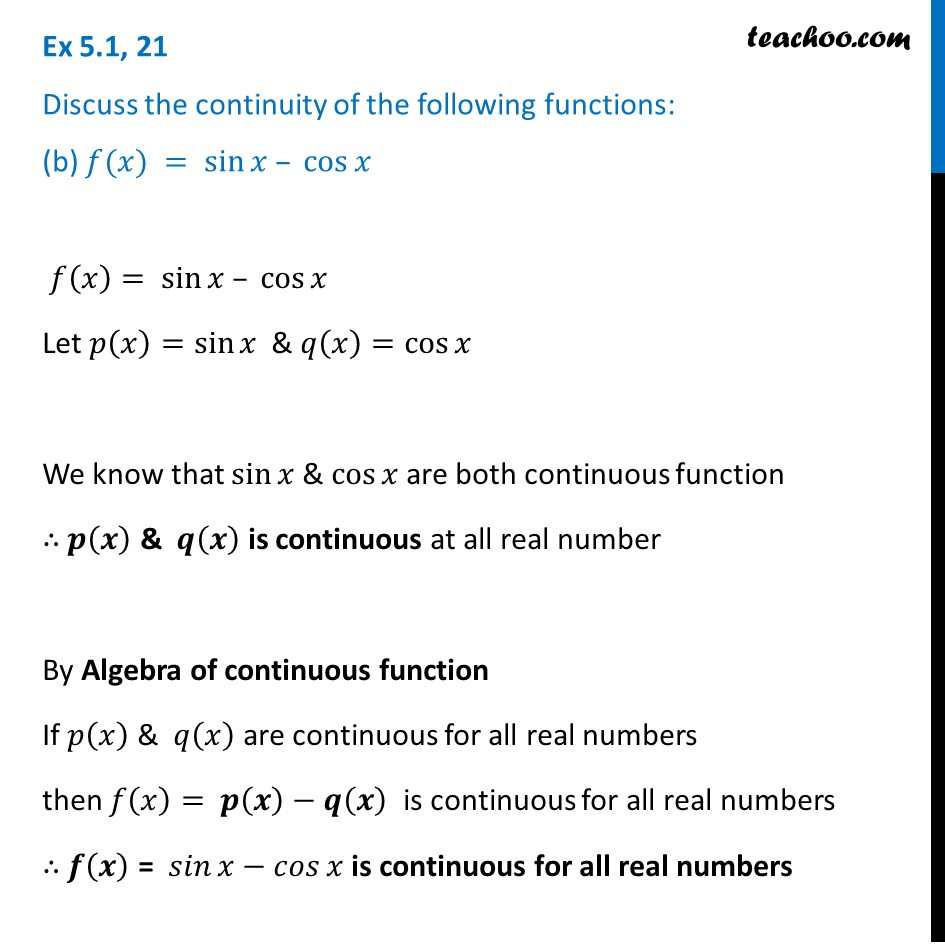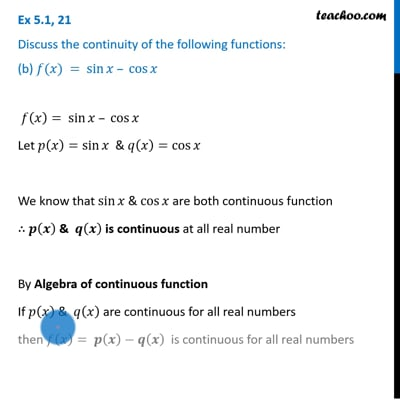This video is only available for Teachoo black users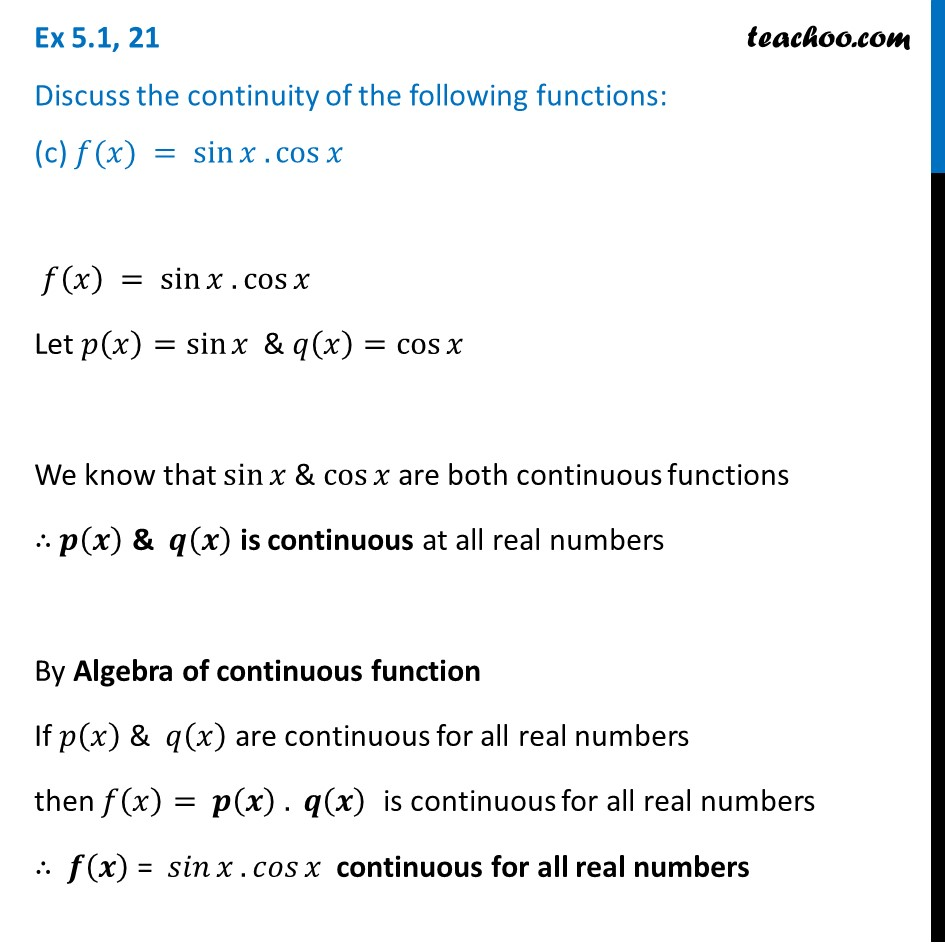Solve all your doubts with Teachoo Black (new monthly pack available now!)

### Transcript

Ex 5.1, 21 Discuss the continuity of the following functions: (a) 𝑓 (𝑥) = sin⁡𝑥+cos⁡𝑥 𝑓 (𝑥) = sin⁡𝑥+cos⁡𝑥 Let 𝑝(𝑥)=sin⁡𝑥 & 𝑞(𝑥)=cos⁡𝑥" " We know that sin⁡𝑥 & cos⁡𝑥 both continuous function ∴ 𝒑(𝒙) & 𝒒(𝒙) is continuous at all real number By Algebra of continuous function If 𝑝(𝑥)" & " 𝑞(𝑥) are continuous for all real numbers then 𝑓(𝑥)= 𝒑(𝒙)+𝒒(𝒙) is continuous for all real numbers ∴ 𝒇(𝒙) = sin⁡𝑥+𝑐𝑜𝑠⁡𝑥 continuous for all real numbers Ex 5.1, 21 Discuss the continuity of the following functions: (b) 𝑓(𝑥) = sin⁡𝑥 – cos⁡𝑥 𝑓(𝑥)= sin⁡𝑥 – cos⁡𝑥 Let 𝑝(𝑥)=sin⁡𝑥 & 𝑞(𝑥)=cos⁡𝑥" " We know that sin⁡𝑥 & cos⁡𝑥 are both continuous function ∴ 𝒑(𝒙) & 𝒒(𝒙) is continuous at all real number By Algebra of continuous function If 𝑝(𝑥)" & " 𝑞(𝑥) are continuous for all real numbers then 𝑓(𝑥)= 𝒑(𝒙)−𝒒(𝒙) is continuous for all real numbers ∴ 𝒇(𝒙) = 𝑠𝑖𝑛⁡𝑥−𝑐𝑜𝑠⁡𝑥 is continuous for all real numbers Ex 5.1, 21 Discuss the continuity of the following functions: (c) 𝑓(𝑥) = sin⁡𝑥 . cos⁡𝑥 𝑓(𝑥) = sin⁡𝑥 . cos⁡𝑥 Let 𝑝(𝑥)=sin⁡𝑥 & 𝑞(𝑥)=cos⁡𝑥" " We know that sin⁡𝑥 & cos⁡𝑥 are both continuous functions ∴ 𝒑(𝒙) & 𝒒(𝒙) is continuous at all real numbers By Algebra of continuous function If 𝑝(𝑥)" & " 𝑞(𝑥) are continuous for all real numbers then 𝑓(𝑥)= 𝒑(𝒙) . 𝒒(𝒙) is continuous for all real numbers ∴ 𝒇(𝒙) = 𝑠𝑖𝑛⁡𝑥.𝑐𝑜𝑠⁡𝑥 continuous for all real numbers